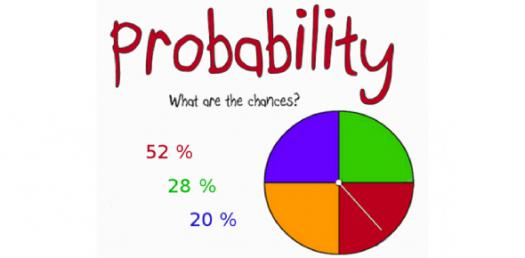# Quiz: Check How Much You Can Score In The Probability Test?

16 Questions | Total Attempts: 259SettingsFor what is the probability used? Probability is a method used to predict the likelihood of uncertain outcomes. It is imperative for the field of genetics because it is used to reveal traits that are hidden in the genome by dominant alleles. Professionals in the medical field make great use of probabilities as do those in agriculture. Probability helps them with the breeding of livestock and weather predictions. This quiz will tell you what you know.

• 1.
If George is rolling one die, what is the probability that he will roll a 6?
• A.

6

• B.

1/6

• C.

1/3

• D.

6/6

• E.

None of the above

• 2.
If a bag contains 3 white marbles, 4 red marbles, and 5 blue marbles, what is the probability of selecting a red marble?
• A.

4

• B.

2/3

• C.

1/3

• D.

4/11

• E.

None of the above

• 3.
If a bag contains 3 white marbles, 4 red marbles, and 5 blue marbles, what is the probability of selecting a red or a blue marble?
• A.

8

• B.

3/4

• C.

1/4

• D.

1/9

• E.

None of the above

• 4.
If a bag contains 3 white marbles, 4 red marbles, and 5 blue marbles, what is the probability of selecting a red marble and then selecting a blue marble without replacement?
• A.

5/33

• B.

7/60

• C.

5/132

• D.

7/132

• E.

None of the above

• 5.
An identity card is picked at random. What is the probability that the sum of the last two digits of its number is 9?
• A.

0.5

• B.

0.4

• C.

0.1

• D.

0.2

• 6.
A box contains 36 marbles. If a marble is picked at random, the probability of being red is 2/9. How many red marbles should be added to make this probability 1/3?
• A.

6

• B.

4

• C.

12

• D.

9

• 7.
The letters of the name SMISS are arranged in a line. If an arrangement is chosen at random, what is the probability that the three Ss are together?
• A.

0.6

• B.

0.3

• C.

0.1

• D.

0.4

• 8.
One of the 5 points (3, 2), (2, 1), (1, - 4), (5, 5) and (4, 6) is selected at random. What is the probability that it lies on the straight line 3x – 2y = 5?
• A.

0.4

• B.

0.2

• C.

0.1

• D.

0.5

• 9.
The letters of the word “PROBABILITY” are written on cards and the cards are then shuffled. If a card is picked at random, find the probability that it will contain a vowel.
• A.

5/11

• B.

3/11

• C.

7/11

• D.

4/11

• 10.
I have two 10-cent coins, three 20-cent coins, four 50-cent coins and five \$1 coins in my pocket. If I choose a coin at random, find the probability that the coin is worth at least 50 cents.
• A.

4/7

• B.

3/7

• C.

9/14

• D.

5/14

• 11.
A man tosses two fair dice. One is numbered 1 to 6 in the usual way and the other is numbered 1, 3, 5, 7, 9 and 11. Find the probability that the total of the two numbers shown is greater than 10.
• A.

7/12

• B.

5/12

• C.

1/2

• D.

1/4

• 12.
A card is drawn at random from a normal pack of 52 cards. If A represents the event that the card drawn is a Queen and B represents the event that the card drawn is a Heart. Find P(A|B).
• A.

5/13

• B.

1/4

• C.

4/13

• D.

1/52

• 13.
Two fair dice, one red and one blue, are tossed. What is the probability that the total of the numbers shown by the two dice exceeds 3?
• A.

1/3

• B.

1/4

• C.

11/12

• D.

1/12

• 14.
A bag contains 30 balls. The balls are numbered 1,2,3,4 ….30. A ball is drawn at random. Find the probability that the number on the ball is a prime number.
• A.

8/9

• B.

2/3

• C.

1/3

• D.

4/9

• 15.
An interview with 18 people revealed that 5 of the 8 women and 8 of the 10 men preferred drinking coffee to tea. What is the probability that if one person is selected from the group of 18 people, it is either a woman or someone who preferred to drink coffee than tea?
• A.

8/9

• B.

1/3

• C.

1/9

• D.

2/3

• 16.
A computer generates 4-digit random numbers in the range 0000 to 9999 inclusive. Find the probability that the computer produces a random number that begins and ends with the digit 1.
• A.

1/10

• B.

1/4

• C.

1/1000

• D.

1/100

Related TopicsBack to top